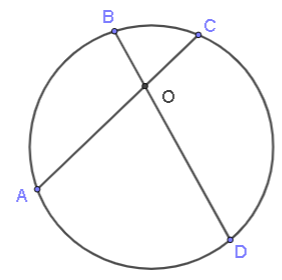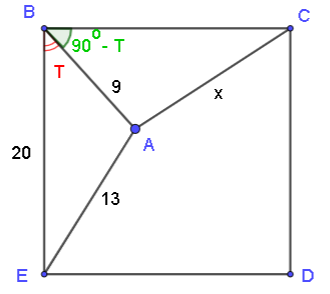# Geometry Problems with Solutions and Answers

Grade 11 geometry problems with detailed solutions are presented.

## Problems

1. Find all points of intersections of the circle x2 + 2x + y2 + 4y = -1 and the line x - y = 1
2. Find the area of the triangle enclosed by the x - axis and the lines y = x and y = -2x + 3.
3. Find the length of the third side of a triangle if the area of the triangle is 18 and two of its sides have lengths of 5 and 10.
4. In the figure below points A, B, C and D are on a circle. Point O is the intersection of chords AC and BD. The area of triangle BOC is 15; the length of AO is 10 and the length of OB is 5. What is the area of triangle AOD?.
5. The two circles below are concentric (have same center). The radius of the large circle is 10 and that of the small circle is 6. What is the length of the chord AB?.
6. Point A is inside the square BCDE whose side length is 20. The length of AB is 9 and the length of AE is 13. Find x the length of AC..

## Solutions to the Above Problems

1. Solve x - y = 1 for x (x = 1 + y) and substitute in the
equation of the circle to obtain:
(1 + y)2 + 2·(1 + y) + y2 + 4y = -1
Expand, group like terms and write the above quadratic equation in standard form
2 y 2 + 8 y + 4 = 0
and solve it to obtain
y = - 2 + √2 and y = - 2 - √2
Use x = 1 + y to find the coordinate x
Points of intersection: ( -1 + √2, - 2 + √2 ) and ( -1 - √2 , -2 - √2 )

2. We first graph the lines y = x and y = -2x + 3 in order to locate the points of intersection of the lines and the x axis and identify the triangle in question..

The height is the y coordinate of the point of intersection of the lines y = x and y = -2x + 3 found by solving the system of equations.
solve : y = -2x + 3 , y = x , solution: (1 , 1) which also the point of intersection. The y coordinate = 1 and is also the height of the triangle.
The length of the base is equal to the x intercept of the line y = -2x + 3 which is x = 3/2.
Area of the shaded triangle = (1/2)(1)(3/2) = 3/4

3. The sine formula for the area using two sides and the internal angle they make, may be written as follows
18 = (1/2) × 5 × 10 × sin(A)
which gives: sin(A) = 18/25
We now use the cosine law to fin the length x of the third side opposing angle A as follows:
x2 = 52 + 102 - 2 × 5 × 10 × cos(A)
with cos(A) = √(1 - sin(A)2)
Substitute in the expression for x2 and solve for x to obtain x = 7.46 (approximated to 3 significant digits)

4. The area of triangle BOC is 15 and is given by (1/2)BO × OC × sin(BOC)
The area of triangle AOD is given by (1/2)AO × OD × sin(AOD)
Note that angle BOC and AOD are equal.
By the theorem of the intersecting chords we have: AO × OC = BO × OD
Which may be written as: AO / BO = OD / OC = 10 / 5 = 2
The ratios AO / BO and OD / OC are both equal to 2, hence their product is equal to 4 as follows
(AO × OD) / (BO × OC) = 4
Which gives: AO × OD = 4 × (BO × OC)
Hence the area of triangle AOD is 4 times the area of triangle BOC and is equal to 60.5. If we draw a radius in the small circle to the point of tangency, it will be at right angle with the chord.(see figure below). If x is half the length of AB, r is the radius of the small circle and R the radius of the large circle then by the Pythagorean theorem we have:
r2 + x2 = R2
62 + x2 = 102
Solve for x: x = 8
Length of AB = 2x = 16.

6. Use cosine law in triangle ABE:
132 = 202 + 92 - 2(20)(9)cos(T)     (I)
Use cosine law in triangle ACB:
x2 = 202 + 92 - 2(20)(9)cos(90° - T).
Note that cos(90° - T) = sin(T) and rewrite the second equation as
Use cosine law in triangle ACB:
x2 = 202 + 92 - 2 (20) (9) cos(90 - T)
Substitute cos(90° - T) = sin(T) to obtain
x2 = 202 + 92 - 2 (20) (9) sin(T)     (III)
Solve the equation (I) for cos(T).
cos(T) = 13/15
Use trigonometric identity sin(T) = √ (1 - cos2 T) to find
sin(T) = 2 √(14) / 15
Substitute sin(T) by 2 √(14) / 15 in equation (III) and solve for x
x = √( 481 - 48 √( 14 ) ) = 17.4 (approximated to 3 significant digits)